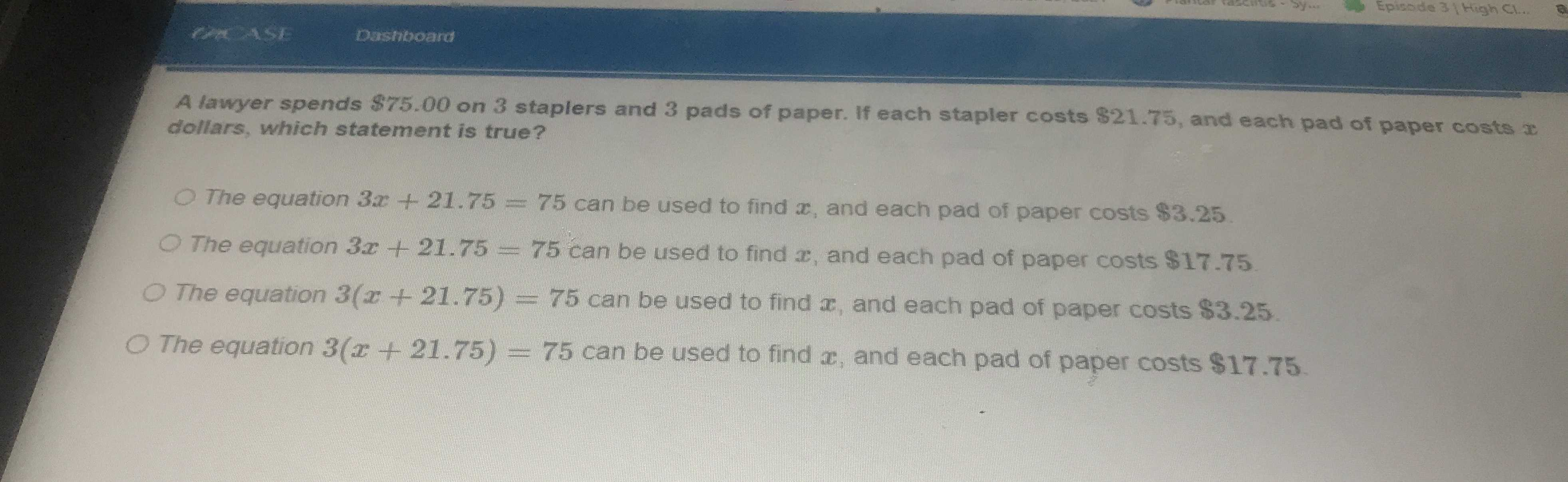### ¿Todavía tienes preguntas de matemáticas?

Pregunte a nuestros tutores expertos
Algebra
PreguntaA lawyer spends $$\ 75.00$$ on $$3$$ staplers and $$3$$ pads of paper. If each stapler costs $$\ 21.75$$ , and each pad of paper costs $$x$$ dollars, which statement is true?

The equation $$3 x + 21.75 = 75$$ can be used to find $$x$$ , and each pad of paper costs $$\ 3.25$$ . The equation $$3 x + 21.75 = 75$$ can be used to find $$x$$ , and each pad of paper costs $$\ 17.75$$. The equation $$3 ( x + 21.75 ) = 75$$ can be used to find $$x$$ , and each pad of paper costs $$\ 3.25$$ .

Then $$3 ( x + 21.75 ) = 75$$ can be used to find $$x$$ , and each pad of paper costs $$\ 17.75$$ .